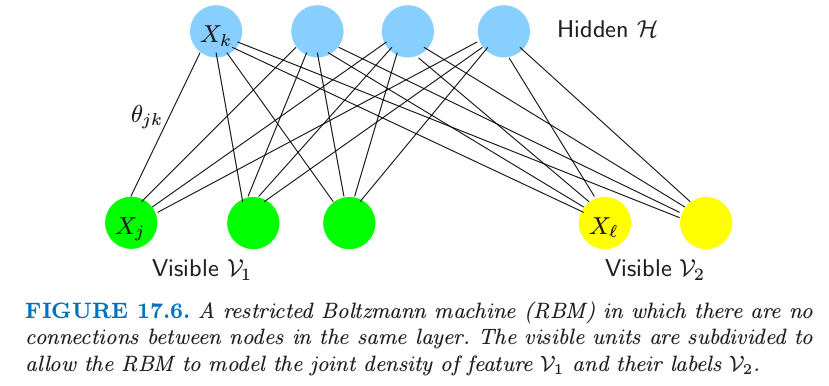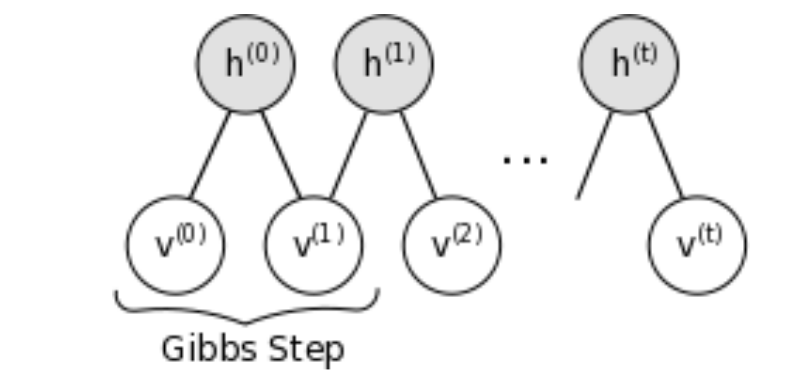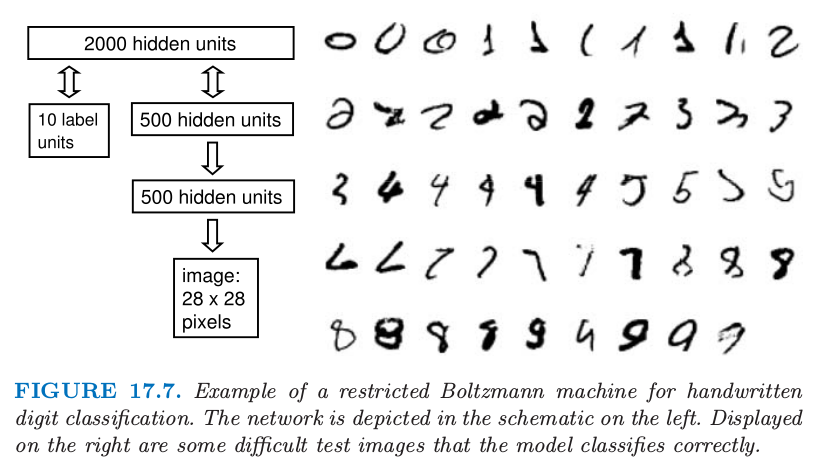17.4 离散变量的无向图模型¶

weiya 注：Recall

weiya 注：Ex. 17.10

weiya 注：multiway tables of counts

Agresti (2002) 在 p17 介绍到，如果一个 列联表 (contigency table) 对两个（类别型）变量进行交叉分类，则称为 two-way table；如果对三个变量交叉分类，则称为 three-way table，以此类推.

Ising 模型暗示着每个结点在其它结点条件下有逻辑斯蒂形式（练习 17.11 其中 $X_{-j}$ 记除了 $j$ 的所有结点．因此参数 $\theta_{jk}$ 衡量了在给定其它结点条件下，$X_j$ 在 $X_k$ 上的依赖性．

weiya 注：Ex. 17.11

当图结构已知时估计参数¶

weiya 注：$2^{p-2}$

• 泊松对数线性建模 (Poisson log-linear modeling)， 其中我们将问题看成是大规模回归问题（练习 17.12）．响应变量 $\mathbf y$ 是数据的多路表的每个单元中的 $2^p$ 维计数的向量．

weiya 注：Ex. 17.12

• 梯度下降 (Gradient descent) 至多需要 $O(p^22^{p-2})$ 的计算量来计算梯度，但是可能会比二阶牛顿法需要更多的梯度步数．然而，可以处理稍微大点的 $p\le 30$ 的问题．计算量可以通过（采用 junction-tree 算法）找出稀疏图中特别的团结构来降低．这里没有给出细节．

• 迭代比例过滤 (Iterative proportional fitting, IPF) 在梯度式 \eqref{17.34} 中采用 循环坐标下降 (cyclical coordinate descent)．每一步更新一个参数使得梯度等式正好为 0．循环进行直到所有梯度为 0．一个完整的周期与梯度赋值花费同样的计算量，但是可能更有效．Jirouśek and Přeučil (1995)4 采用 junction trees 实现了 IPF 的一个有效版本．

• 均值域近似（Peterson and Anderson, 19875）用 $\E_{\mathbf\Theta}(X_j)\E_{\mathbf \Theta}(X_k)$ 来估计 $\E_{\Theta}(X_jX_k)$，并且将输入变量用它们的均值替换，得到一系列关于参数 $\theta_{jk}$ 的非线性等式．
• 为了得到近似解，吉布斯采样（8.6 节）可以用来近似 $\E_{\mathbf\Theta}(X_jX_k)$，即对估计模型 $\Pr_{\mathbf\Theta}(X_j\mid X_{-j})$ 逐步抽样（见 Ripley (1996)6

隐藏结点¶

weiya 注：推导 \eqref{17.38}

图结构的估计¶

Lee et al. (2007)7 和 Wainwright et al. (2007)8 建议使用二值成对马尔科夫网络的 lasso 惩罚．第一篇作者们提出共轭梯度法来精确最大化含惩罚的对数似然．瓶颈是梯度中 $\E_{\mathbf\Theta}(X_jX_k)$ 的计算；通过 junction tree 算法的精确计算对于稀疏图是可行的但是对稠密的图变得难以处理．

限制玻尔兹曼机¶

weiya 注：weiya 注截图自http://deeplearning.net/tutorial/1. Bishop, Y., Fienberg, S. and Holland, P. (1975). Discrete Multivariate Analysis, MIT Press, Cambridge, MA.

2. McCullagh, P. and Nelder, J. (1989). Generalized Linear Models, Chapman and Hall, London.

3. Agresti, A. (1996). An Introduction to Categorical Data Analysis, Wiley, New York.

4. Jirouśek, R. and Přeučil, S. (1995). On the effective implementation of the iterative proportional fitting procedure, Computational Statistics and Data Analysis 19: 177–189.

5. Peterson and Anderson, J. R. (1987). A mean field theory learning algorithm for neural networks, Complex Systems 1: 995–1019.

6. Ripley, B. D. (1996). Pattern Recognition and Neural Networks, Cambridge University Press.

7. Lee, S.-I., Ganapathi, V. and Koller, D. (2007). Efficient structure learning of markov networks using l 1 -regularization, in B. Schölkopf, J. Platt and T. Hoffman (eds), Advances in Neural Information Processing Systems 19, MIT Press, Cambridge, MA, pp. 817–824.

8. Wainwright, M. J., Ravikumar, P. and Lafferty, J. D. (2007). High-dimensional graphical model selection using l 1 -regularized logistic regression, in B. Schölkopf, J. Platt and T. Hoffman (eds), Advances in Neural Information Processing Systems 19, MIT Press, Cambridge, MA, pp. 1465–1472.

9. Meinshausen, N. and Bühlmann, P. (2006). High-dimensional graphs and variable selection with the lasso, Annals of Statistics 34: 1436–1462.

10. Hoefling, H. and Tibshirani, R. (2008). Estimation of sparse Markov networks using modified logistic regression and the lasso, submitted.

11. Hinton, G. (2002). Training products of experts by minimizing contrastive divergence, Neural Computation 14: 1771–1800.

12. Le Cun, Y., Bottou, L., Bengio, Y. and Haffner, P. (1998). Gradient-based learning applied to document recognition, Proceedings of the IEEE 86(11): 2278–2324.

13. Hinton, G., Osindero, S. and Teh, Y.-W. (2006). A fast learning algorithm for deep belief nets, Neural Computation 18: 1527–1554. 下载